Please enable Javascript for your browser if it supports it or use the Browser that supports Javascript.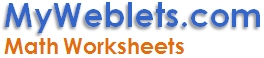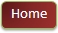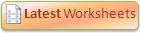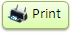Hide Answer Key Want to get notified about new worksheets?

 www.MyWeblets.com/kids/math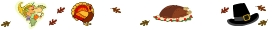Math Worksheet #859 (L-G2)
 Name:_____________________ Date: __________ Start Time: _________ Finish Time: _________

Buy each pumpkin with fewest coins and total the number of coins.
Color the pumpkin that need the least number of coins.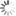Loading! Please Wait ...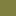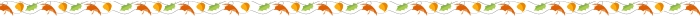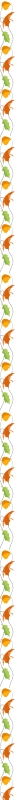Hint: Use bigger coins first and then the smaller coins.25 ¢10 ¢5 ¢1 ¢ Total coins EXAMPLE 1 1 0 4 6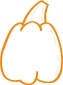39 ¢ Hint: Start with 1 quarter to make the total 25 ¢. You can't add more quarters as the total will become 50 ¢ (25  ¢ + 25 ¢), which is more than the cost of the pumpkin (39 ¢). You have 1 quarter, so write the answer above and move to the smaller coin to make the total 39 ¢. Hint: Add a dime to make the total 35 ¢ (25 ¢ + 10 ¢). You can't add more dimes  as the total will become 45 ¢ (35  ¢+ 10 ¢), which is more than the cost of pumpkin (39 ¢). You have 1 dime, so write the answer above and move to the smaller coin to make the total 39 ¢. Hint: You can't add a nickel as the total will become 40 ¢ (35  ¢+ 5 ¢), which is more than the cost of pumpkin (39 ¢). You have 0 nickels, so write the answer above and move to the smaller coin to make the total 39 ¢. Hint: This is the smallest coin, so add as may pennies as required to make the final total. For this question add 4 pennies to make the total 39 ¢ (35 ¢ + 4 ¢) Hint: Count all the coins and write you answer above. 3 + 2 + 4 = 9 Try yourself51 ¢ _____ _____ _____ _____ _____99 ¢ _____ _____ _____ _____ _____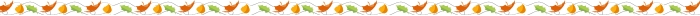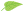Answer Key: (Cut the Answer Key or Fold It) Use "Hide Answer Key" option at the top if needed. (1) 6 (2) 3 (3) 9